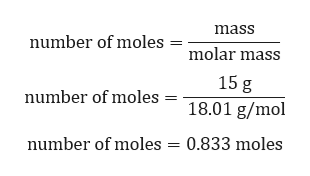# An electrical heater is used to supply 210.0 J of energy to a 15.0 g sample of water, originally at 295.0 K. Determine the final temperature in degrees Kelvin. (the specific heat capacity for water is 75.291 Jmol-1K-1)

Question
2 views

An electrical heater is used to supply 210.0 J of energy to a 15.0 g sample of water, originally at 295.0 K. Determine the final temperature in degrees Kelvin. (the specific heat capacity for water is 75.291 Jmol-1K-1)

check_circle

Step 1

Given:

Heat energy (Q) = 210.0 J.

Mass of water (m) = 15.0 g.

Initial temperature of water = 295 K.

Specific heat capacity for water (C) = 75.291 Jmol-1K-1

Step 2

As, specific heat is given in J/mol.K. so mass should be converted to moles.

Calculation for number of moles of water:help_outlineImage Transcriptionclosemass number of moles molar mass 15 g 18.01 g/mol number of moles = number of moles = 0.833 moles fullscreen
Step 3

Energy needed to raise the te...

### Want to see the full answer?

See Solution

#### Want to see this answer and more?

Solutions are written by subject experts who are available 24/7. Questions are typically answered within 1 hour.*

See Solution
*Response times may vary by subject and question.
Tagged in

### Chemistry